# Adding the Power Fx Formula column (PREVIEW) into Dataverse

By | August 24, 2022

Recently when I was adding the new column in Microsoft Dataverse from the PREVIEW maker portal (https://make.preview.powerapps.com/) I was amazed by looking at the new option in the Datatype available named “Formula”.

In this blog, we will focus on the introduction and related use cases where this “Formula” datatype can be useful.

Formula Column Features:

• Formula columns use the Power Fx syntax similar to Office Excel. Power Fx is the low code language that is being used across the Microsoft Platform.
• Formula column expands the ability of existing calculated and rollup columns it adds the value in expression by performing the arithmetic calculation in real-time.
• The formula expression can be made available with Today () and Now () functions for the first time.
• Many supported functions are available for inbuilt arithmetic operations.
• As you enter the formula, Intellisense helps you with recommendations for formula, syntax, and errors.
• Making it easy to see and edit the column’s formula directly inline without needing to save or open another window as we do for calculated and rollup fields where another window opens up for entering the calculations.

Adding the “Formula” column in Dataverse:

Pre-requisite:

• You must navigate to the PREVIEW maker portal (https://make.preview.powerapps.com/) and under the appropriate environment try to add the column by using the steps explained in the below use cases.

Use Case1:

• Suppose there is a requirement to compare the “Sprint Review Meeting Date” (Time Zone Independent date) with today’s date and if the difference between these two days is less than or equal to 7, then set the value in “Sprint Retrospective Decision” field as either “Sprint Goal achieved” or “Sprint Goal not achieved”.
• Earlier to achieve this requirement we would have had to go with custom logic development (e.g. Power Automate etc.) but with the introduction of the “Formula” column in Dataverse, it is now easy to implement this logic by using appropriate functions.

Implementation:

• You can implement the above requirement using UTCTODAY and DateDiff inbuilt functions.
• As you can see in the following screenshot, you can use the formula datatype to create a formula column that computes arithmetic operations by incorporating the inbuilt functions.

Steps:

1. Select the table where you want to add the column. For this demonstration, I have used the “Sprint Evaluation” custom table.

2. Click on “+ New Column” and in the Add new column pane, select “Formula” as the data type. As shown below I have added the “Sprint Retrospective Decision” column: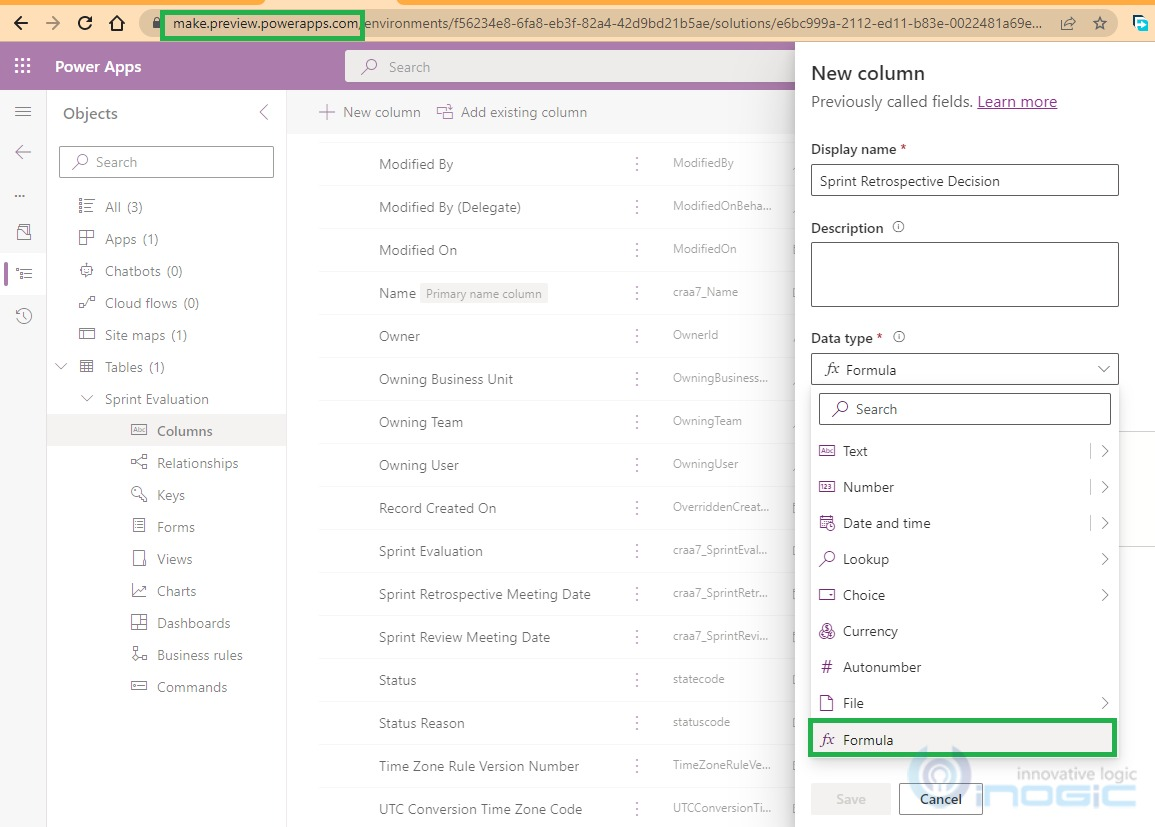3. Upon selecting the “Formula” datatype, the expression box opens up where you can enter the desired formula.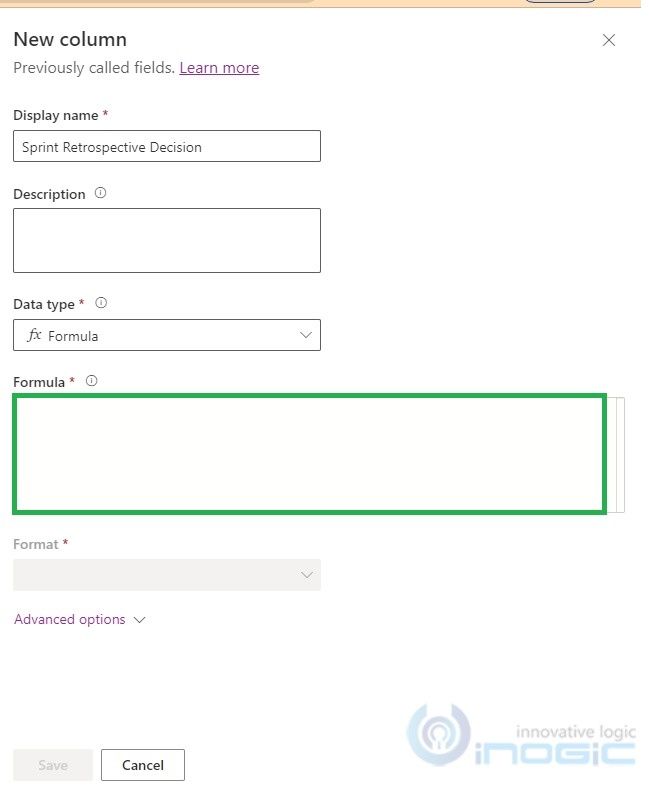4. For the demonstration, implemented formula expression is as shown: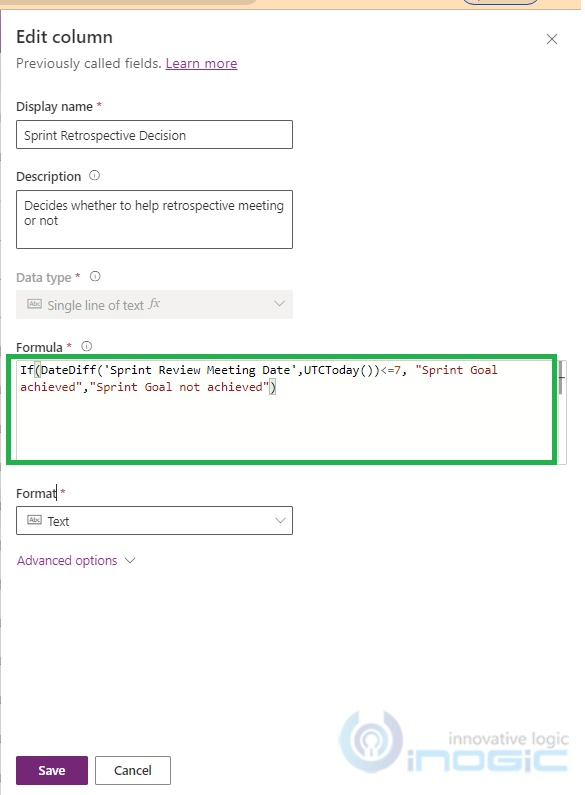Formula Expression used:

• If(DateDiff(‘Sprint Review Meeting Date’,UTCToday())<=7, “Sprint Goal achieved”,”Sprint Goal not achieved”)

UI Demonstration:

• While creating “Sprint Evaluation” record or on change of “Sprint Review Meeting Date” the value in “Sprint Review Meeting Date” is compared with the “today’s date”. As difference between these dates is less than 7, “Sprint Retrospective Decision” is set as “Sprint Goal achieved” as shown in the below screenshot of the model-driven app: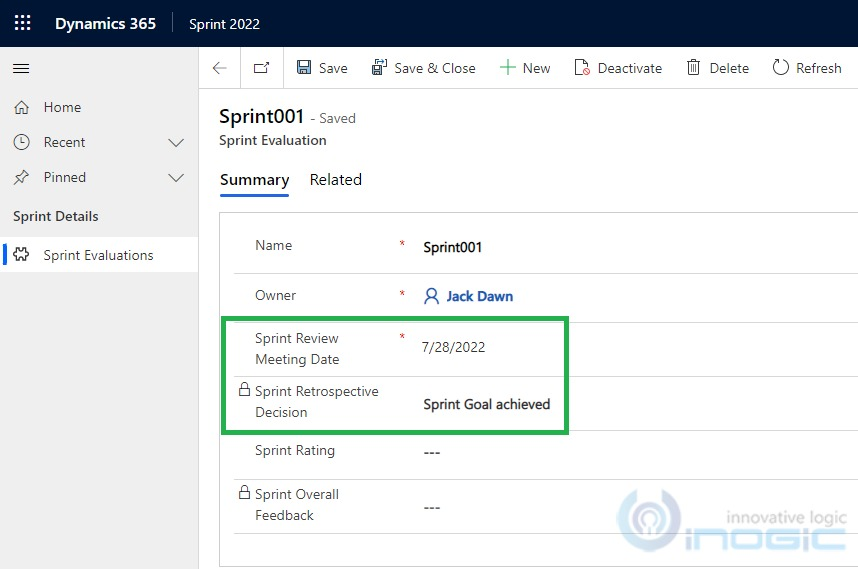Use Case 2:

• Suppose there is a requirement to check the value entered in the “Sprint Rating”. Thus, depending upon the value entered, set “Sprint Overall Feedback” as tabulated below:• Earlier to achieve this requirement we had to go with custom logic development (e.g. Power Automate etc.) but with the introduction of the “Formula” column in Dataverse, it is now easy to implement this logic by using appropriate inbuilt functions.

Implementation:

• You can implement the above requirement using Switch inbuilt functions.
• As you can see in the following screenshot, you can use the formula datatype to create a formula column that computes arithmetic operations by incorporating the function.

Steps:

1. Select the table where you want to add the column. For this demonstration, I have used the “Sprint Evaluation” custom table.

2. Click on “+ New Column” and in the Add new column pane, select “Formula” as the data type. As shown below I have added the “Sprint Overall Feedback” column: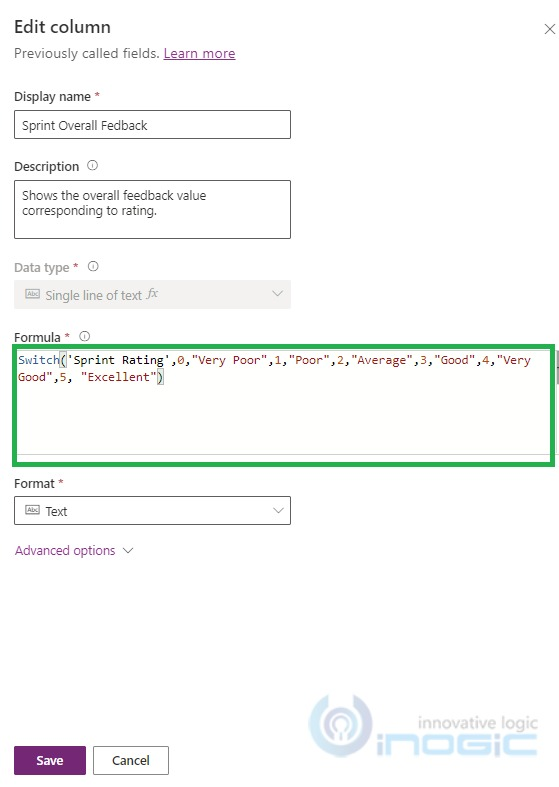Formula Expression used:

• Switch(‘Sprint Rating’,0,”Very Poor”,1,”Poor”,2,”Average”,3,”Good”,4, “Very Good”,5, “Excellent”)

UI Demonstration:

• While creating “Sprint Evaluation” record or on change of “Sprint Rating” the value in “Sprint Overall Feedback” is set according to above explained logic. Let’s say, the “Sprint Rating” is entered as 5 and the “Sprint Overall Feedback” is set as Excellent as shown in below screenshot of the model driven app: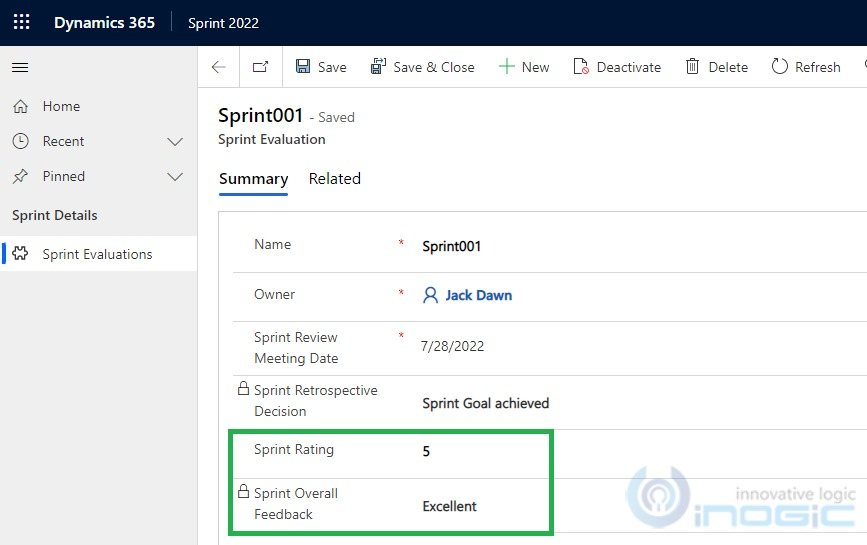While exploring I liked the real time troubleshooting and real time refresh that it offers, as explained below:

• The formula editor supports IntelliSense to suggest formulas and errors in real time: For e.g. If while building the formula expression double quote (“) missed in the real time then on hovering the mouse above it, it will show the error description as below: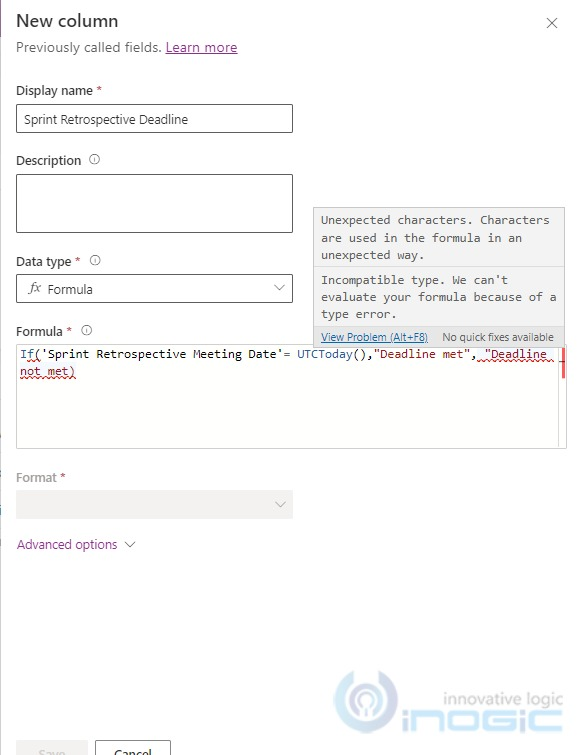• The formula editor supports instant refresh in real time: When you create a record within the model drive app, the formula column executes the defined formula expression and displays the data for the record instantly without refreshing the record.

You can find more details in this doc where you can find the minimum limitations experienced as well, while working with the Formula column remember this feature is still in PREVIEW.

## Conclusion

The Power Fx formula provides a richer and easier experience while using the column calculations in Microsoft Dataverse which is also progressing to the next level of adding columns seamlessly with supported inbuilt functions.

## Save 1-2 hours or \$800 monthly on scheduling and managing business travel with a geo-mapping App!

Maplytics – Integrate Map with Dynamics 365 CRM and visualize data on the map, manage sales territories, auto-schedule appointments, get optimized travel routes, track field reps in real-time, and more